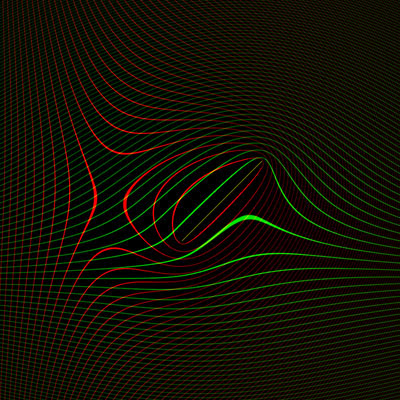## Monday, May 28, 2007

### Pathfinding in Space, part 3

Last week I ended with the vector equation I've been stuck trying to solve. vm is the unknown:The equation describes accelerating at a constant rate of 1 from a starting velocity v0 to an intermediate velocity vm, and then at the same rate of 1 (but possibly in a different direction) from vm to a final velocity vf. The desired displacement resulting from this trip is r.

Essentially we have position and velocity endpoint conditions, and a limit on how fast we can accelerate. My hypothesis is that the quickest path connecting the endpoints will be two parabolas (with the max acceleration on each) that fit the ends and match position and velocity where they meet.

I will probably put this problem on the back burner for a bit, but before doing so I thought I'd at least produce some graphs to get a better feel for it. I wrote a quick program that uses OpenGL to produce graphs of velocity space.

The program takes in a v0 and vf pair. Each point on the graph is a candidate vm; the program uses it to evaluate the right-hand side of the equation to give the r corresponding to that solution.

Lines of constant rx are drawn in red and lines of constant ry in green. If they were labeled, you could find a rough solution by locating the red line and green line that correspond to the desired r, and then locating an intersection between them. The position of the intersection is the value for vm that solves the equation.

Here is the simplest case, where the starting and ending velocities are both zero:(0, 0) to (0, 0)

The program dices the screen up into squares a few pixels on a side. For each square, it evaluates r at the four corners, then draws two textured quads additively over it. The first is drawn in red and uses rx as the Z coordinate. I've set OpenGL to map the Z value into a repeating one-dimensional texture which contains a few white pixels followed by a bunch of black ones. This creates the periodic lines. The texture is mipmapped to cut down on aliasing, so when the lines get really thin they fade out rather than dissolving into sparkles. The second quad is drawn in green, using ry as the Z coordinates for the quad corners.

Here's a slightly more complicated example, with starting velocity of zero:(0, 0) to (8, 0)

It looks like there is still a unique solution for every given displacement. The exception to this is on the line from (0, 0) to (8, 0) itself (drawn in yellow and faintly visible over the green); any point on there will give the same r.

Let's mix things up a bit more:(6, -2) to (6, 2)

Now there are closed loops of constant rx. There are some green and red lines that cross each other twice, indicating that for those values of r there are two solutions.

Here's a diagonal movement in velocity space:(5, 0) to (10, 5)

Now there are some red and green lines that cross each other three times! So there are at least some cases that have three solutions. I don't know if there are situations with four solutions. The minimum-time solution is the one closest to v0 and vf.

If nothing else, this would make a really wild screensaver, if you animated v0 and vf to bounce around the screen.

Amit said...

I probably don't understand all the constraints, but I think that in 2d or 3d space, the shortest path is still a straight line, just as in the 1d case. Instead of treating x and y separately, construct a new 1d “space” which is essentially the position u along the straight line from the start to the goal. Then use your 1d solution along this line, and convert back from u coordinates to x,y.

James McNeill said...

I'd love to get back to this project but I've been working on others for quite a while now. Hopefully I'm remembering right.

Even though the movement is in a 2D plane, because velocity is allowed to vary it is a part of the state space too. So the state space for the search has at least four dimensions.

Imagine that we want to get to the same position as where we currently are, but with a different velocity. A cost heuristic that considered only position (and not velocity too) would underestimate the cost for this maneuver, arbitrarily drastically depending on the desired change in velocity. This would result in many more nodes being searched. (Since the search space is four-dimensional it's easy to get an explosion of nodes searched anyway.)

Conversely, let's suppose that we want to get to a position (and velocity) which our current velocity will take us to on the next turn. A cost heuristic that considered only position might overestimate the cost to make this maneuver, which could result in the correct nodes not being searched at all.

Gerry said...

Although in the pathfinding space it is only 2D but you graphically showed the effect of different velocities. https://deanhineslawyer.com/divorce-lawyers-dayton-ohio/

Gerry said...

Can this be done as a screensaver? Just asking. book now